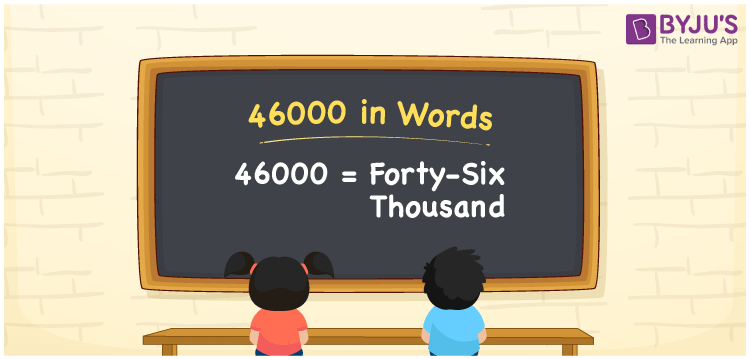# 46000 in Words

The number name for the numerical 46000 is Forty-six Thousand. The number 46000 in words is written using the place value of the given number. Hence, the place value chart plays a major role in writing the number name. For example, if you spent Rs. 46000 on purchasing a laptop, you can write “I spent Rs. Forty-six thousand on purchasing a laptop”. In this article, we are going to discuss the spelling of 46000 in English, and the procedure for writing the number name of 46000 using the place value system in detail.

 46000 in Words: Forty-six Thousand Forty-six Thousand in Numerical Form: 46000

## 46000 in English Words## How to Write 46000 in Words?

Following is the place value chart for the number 46000. The number 46000 in words is written by identifying the place value of the number:

 Ten – Thousands Thousands Hundreds Tens Ones 4 6 0 0 0

The expanded form the number 46000 is as follows:

= 4 × Ten thousand+ 6 × Thousand × 0 × Hundred × 0 × Ten + 0 × One

= 4× 10000 + 6 × 1000 + 0 × 100 + 0 × 10 + 0 × 1.

= 46000

= Forty-six Thousand

Therefore, 46000 in words is Forty-six Thousand.

The natural number 46000 appears before the number 46001 and just after 45999.

46000 in words – Forty-six Thousand

Is 46000 an odd number? – No

Is 46000 an even number? – Yes

Is 46000 a perfect square number? – No

Is 46000 a perfect cube number? – No

Is 46000 a prime number? – No

Is 46000 a composite number? – Yes

## Frequently Asked Questions on 46000 in Words

### How to write 46000 in words?

46000 in words is forty-six thousand.

### Simplify 47000 – 1000, and express in words.

Simplifying 47000 – 1000, we get 46000. Hence, 46000 in words is forty-six thousand.

### How to write forty-six thousand in numbers?

Forty-six thousand in numbers is 46000.